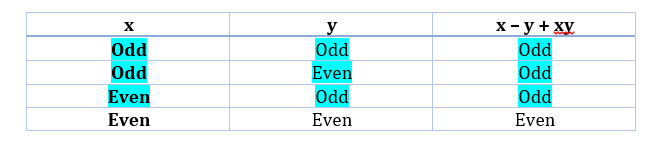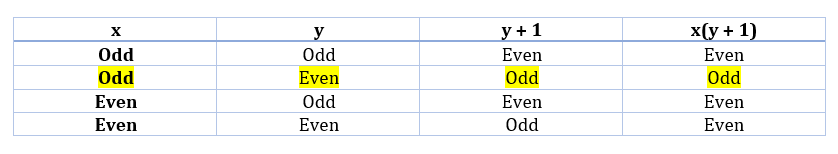Summer is Coming! Join the Game of Timers Competition to Win Epic Prizes. Registration is Open. Game starts Mon July 1st.

 It is currently 21 Jul 2019, 04:39### GMAT Club Daily Prep

#### Thank you for using the timer - this advanced tool can estimate your performance and suggest more practice questions. We have subscribed you to Daily Prep Questions via email.

Customized
for You

we will pick new questions that match your level based on your Timer History

Track

every week, we’ll send you an estimated GMAT score based on your performance

Practice
Pays

we will pick new questions that match your level based on your Timer History

#### Not interested in getting valuable practice questions and articles delivered to your email? No problem, unsubscribe here.# x, y are positive integers. Find the number of even factors of 4x^2

Author Message
TAGS:

### Hide Tags

e-GMAT RepresentativeV
Joined: 04 Jan 2015
Posts: 2943
x, y are positive integers. Find the number of even factors of 4x^2  [#permalink]

### Show Tags

3
1800:00

Difficulty:95% (hard)

Question Stats:27% (02:46) correct73% (02:53) wrongbased on 356 sessions

### HideShow timer StatisticsQuestion 3 of the e-GMAT Primes Trio: 3 Questions on Number of factors and prime factors

$$x, y$$ are positive integers. Find the number of even factors of $$4x^2$$

I. $$x^3 – y^3 + 3xy$$ is odd and x is a prime.
II. $$x^{(x+y)}*y^{3x} +x^{3y}$$ is odd.

Here is a fresh question from e-GMAT. Go ahead and give it a shot!Regards,
The e-GMAT Quant Team

P.S.: Solutions with clarity of thought and elegance will get kudos!Here is an official question which tests similar concept.
http://gmatclub.com/forum/if-n-4p-where ... 44781.html

Hope this helps._________________

Originally posted by EgmatQuantExpert on 05 May 2015, 02:17.
Last edited by EgmatQuantExpert on 07 Aug 2018, 02:55, edited 7 times in total.
e-GMAT RepresentativeV
Joined: 04 Jan 2015
Posts: 2943
Re: x, y are positive integers. Find the number of even factors of 4x^2  [#permalink]

### Show Tags

4
6
Here is the solution for this question.Steps 1 & 2: Understand Question and Draw Inferences

We are given that x and y are positive integers. The question asks us to find the number of even factors of $$4x^2$$

The Number of Even Factors of a number = Total Number of Factors – Number of Odd Factors

To find the total number of factors we need to know the powers of each prime factor.

To find the number of odd factors we need to know the powers of every odd prime factor.

So essentially we need to know the prime factorization of $$4*x^2$$

In other words, we need to know the prime factorization of $$x^2$$ or $$x$$ to be able to answer the given question.Step 3: Analyze Statement 1: $$x^3 – y^3 + 3xy$$ is odd and $$x$$ is a prime.

This statement says $$x$$ is a prime. So the prime factorization of $$4*x^2$$ will be $$2^2 * x^2$$

However, we still don’t know whether $$x$$ is even or odd.

If $$x$$ is even, then $$x = 2$$ which gives us $$4*x^2$$ as $$2^4$$ and since this expression doesn’t have any odd prime factors, the total number of even factors are same as the total number of factors. (which will be $$5$$ in this case.)

However, if $$x$$ is an odd prime, then $$4*x^2$$ will be $$2^2 * x^2$$.

In this case, the total number of factors will be $$(2+1)*(2+1) = 9$$

And the number of odd factors will be $$(2+1) = 3$$

This gives us the number of even factors = $$9 – 3 = 6$$

So we need to know whether $$x$$ is even or odd to determine a unique answer.

We will try to use the other piece of information ” $$x^3 – y^3 + 3xy$$ is odd” to determine the even-odd nature of $$x$$.

We know that a positive integral power doesn’t affect the even-odd nature. Similarly, multiplication by a odd number won’t affect the even-odd nature.

Therefore if $$x^3 – y^3 + 3xy$$ is odd then $$x – y + xy$$ must also be odd. So let us now see the various cases where $$x – y + xy$$ must be odd.As you can see, $$x – y + xy$$ is odd when $$x$$ is odd and also in a case when $$x$$ is even.

So we cannot determine whether $$x$$ is even or odd.

Therefore statement 1 is not sufficient to arrive at a unique answer.

Step 4: Analyze Statement 2: $$x^{(x+y)}*y^{3x} +x^{3y}$$ is odd.

Since positive integral powers do not affect the even-odd nature, statement 2 essentially tells us that $$xy + x$$ is odd. Which means $$x*(y+1)$$ is odd. So let us now see the various cases where $$x*(y + 1)$$ must be odd.As you can see, “$$x(y + 1)$$ is odd” implies that “$$x$$ is odd”.

However, $$x$$ need not be a prime. In other words, $$x$$ could be written as $$x = p^a * q^b * r^c$$ … where $$p$$, $$q$$, $$r$$, etc. are primes and $$a$$, $$b$$, $$c$$ are non-negative integers. Therefore without knowing these values we cannot determine the prime factorization of $$x$$ and therefore cannot arrive at a unique answer.

Therefore statement 2 is not sufficient.

Step 5: Analyze Both Statements Together (if needed):

From statement 1, we have: $$x$$ is a prime and we need to know if $$x$$ is even or odd to arrive at a unique answer.

From statement 2, we have: $$x$$ is odd.

Therefore both statements together are sufficient.

Hope this helps.Regards,
Krishna
_________________

Originally posted by EgmatQuantExpert on 05 May 2015, 02:18.
Last edited by EgmatQuantExpert on 08 May 2015, 07:18, edited 1 time in total.
##### General Discussion
ManagerJoined: 18 Nov 2013
Posts: 78
Concentration: General Management, Technology
GMAT 1: 690 Q49 V34Re: x, y are positive integers. Find the number of even factors of 4x^2  [#permalink]

### Show Tags

1
Q : x,y are positive integers. Find the number of even factors of 4$$x^{2}$$

from the question stem we need to find out factors of 4$$x^{2}$$ and then pick up even factors , essentially we need two things value of x (is it a composite number) and weather x is odd/even.

4$$x^{2}$$ == > $$2^{2}$$$$x^{2}$$

Note: if x is odd , even factors will be less (only the ones with multiple of 2 will be counted)

Stmt I. $$x^{2}$$-$$y^{3} +3xy$$ is odd and x is a prime.

x is prime so that means (2, x) are the only prime factors of $$4x^{2}$$ = $$2^{2}$$$$x^{2}$$ ==> can have (2+1)(2+1) = 9 factors
how many are even ?
x is prime can be 2, 3 (if its odd i.e prime $$\neq{2}$$ ) , then we can figure out (even factors out of 9 factors)

$$x^{2}$$-$$y^{3}$$ +3xy = odd

x - y + 3xy = odd (power does not matter in calculation odd/even nature)

x -> even , then y -> odd for above equation to be true
x -> odd , then y -> even for above equation to be true

x can be odd prime or even prime, so Not Sufficient

Stmt II. $$x^{x+y}$$∗$$y^{3x}$$+$$x^{3y}$$ is odd.

this stmt only tells us about the even/odd nature (so won't be sufficient in determine the actual answer)

$$x^{x+y}$$∗$$y^{3x}$$+$$x^{3y}$$ = odd.

xy + x = odd (power does not matter in calculation odd/even nature)

x(y + 1) = odd

from above x is odd (x can't be even, y can't be odd)

x is odd but can be (3, 9, 25) so Not Sufficient

combining Stmt I. and Stmt II.

x is odd , and x is prime = Sufficient
4$$x^{2}$$ = $$2^{2}$$$$x^{2}$$ ==> can have (2+1)(2+1) = 9 factors

x is prime and x is odd i.e $$x\neq{2}$$

total even factors = 2,4,2x,4x,2x^2,4x^2...

Ans : C
_________________
_______
- Cheers

+1 kudos if you like
Board of DirectorsP
Joined: 17 Jul 2014
Posts: 2539
Location: United States (IL)
Concentration: Finance, Economics
GMAT 1: 650 Q49 V30GPA: 3.92
WE: General Management (Transportation)
Re: x, y are positive integers. Find the number of even factors of 4x^2  [#permalink]

### Show Tags

i highly doubt a gmat question would be like this. I personally find e-gmat questions waaay tougher than the gmat hard questions...lack of feedback from users just confirms this..
my 2 cents on solving this one..although I got it wrong the first time...because I was spending way to much to solve it >3 mins (~4 min).

EgmatQuantExpert wrote:
$$x, y$$ are positive integers. Find the number of even factors of $$4x^2$$

I. $$x^3 – y^3 + 3xy$$ is odd and x is a prime.
II. $$x^{(x+y)}*y^{3x} +x^{3y}$$ is odd.

x is prime.
so x=even or x=odd.
y - even or odd.
x -even y even
we have 4 cases:
even-even+even=even out.
even-odd+even=odd - works
odd-even+odd = even - out.
odd-odd+odd = odd. - works

x can be even or odd.
since we need to find the prime factorization of x, we cannot solve the question.

2.expression is odd.
we have few cases:
x - odd or even
y - odd or even

x - odd, y even.
odd*even+odd - odd - works
x-odd, y-odd.
odd*odd+odd - even, out.
x-even, y-even.
even*even+even - even - out.
x-even, y-odd
even*odd+even - even, out.

we clearly see that x is odd, and y is even.
alone is not sufficient.

1+2
x is prime, and it is odd.
thus, x^2 will have only 1 prime factor.
suppose x=3.
then, 4x^2 will be broken down to 2^2*3^2, or total number of factors 3*3=9.
odd factors - 2.
even factors - 7.

_________________
ManagerB
Joined: 27 Mar 2014
Posts: 73
Schools: ISB '19, IIMA , IIMB
GMAT 1: 660 Q49 V30Re: x, y are positive integers. Find the number of even factors of 4x^2  [#permalink]

### Show Tags

x,y are positive integers. Find the number of even factors of 4x^2

I. x^3–y^3+3xy is odd and x is a prime.
II. x^(x+y)∗y^(3x)+x^(3y) is odd.

Statement 1 "

X is prime. so, X can be even or odd

x^3–y^3+3xy is odd

Can not find , whether X is even or odd

Insufficient

Statement 2

x^(x+y)∗y^(3x) + x^(3y) is odd.

statement 2 can be odd , in 2 ways

1st way

x^(3y) is even & x^(x+y)∗y^(3x) is odd

x^(3y) is even , then x is even.

As x is even x^(x+y)∗y^(3x) can not be odd.

so we get for x^(x+y)∗y^(3x) + x^(3y) to be odd.

x^(3y) is odd & x^(x+y)∗y^(3x) is even

or x is odd

Insufficient ; different values of odd (X) will result in different even factors for 4x^2

combing st 1 & st 2

X is odd & prime

we get total even factors = 2

Non-Human UserJoined: 09 Sep 2013
Posts: 11719
Re: x, y are positive integers. Find the number of even factors of 4x^2  [#permalink]

### Show Tags

Hello from the GMAT Club BumpBot!

Thanks to another GMAT Club member, I have just discovered this valuable topic, yet it had no discussion for over a year. I am now bumping it up - doing my job. I think you may find it valuable (esp those replies with Kudos).

Want to see all other topics I dig out? Follow me (click follow button on profile). You will receive a summary of all topics I bump in your profile area as well as via email.
_________________Re: x, y are positive integers. Find the number of even factors of 4x^2   [#permalink] 27 Dec 2018, 09:07
Display posts from previous: Sort by

# x, y are positive integers. Find the number of even factors of 4x^2